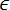# How to insert an equation with integral

Word
This tip display how to insert an equation for example, the Gauss's law, also known as Gauss's flux theorem.

How to add an equation in your document, see Working with Microsoft Equation.

To add integral form of the Gauss's law, do the following:

1.   In the Equation Editor select Contour integral with subscript limit in the Integral templates group on the Equation toolbar:2.   Enter S in the lower box of your integral.

3.   In the base box of the integral enter E and then choose Bullet in the Operator symbols group on the Equation toolbar:4.   Enter dA=.

5.   In the Equation Editor enter f(x)= and then select Full-size vertical fraction in the Fraction and radical templates group on the Equation toolbar:6.   Enter Q in the top of this template and in the bottom:

• Choosein the Greek characters (lowercase) group on the Equation toolbar:• Select Subscript in the Subscript and superscript templates group on the Equation toolbar:• Enter 0 in the lower right box of script.